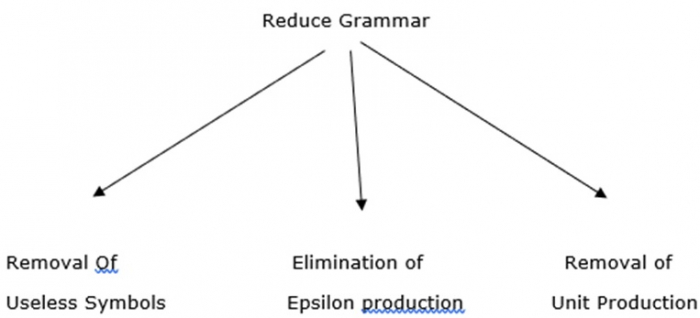# Explain the elimination of epsilon productions in CFG

All grammars are not always optimized, which means the grammar may consist of some extra symbols (non-terminals) which increase the length of grammar.

So, we have to reduce the grammar by removing the useless symbols.

## Properties

The properties to reduce grammar are explained below −

• Each non-terminal and terminal of G appears in the derivation of some word in L.
• There should not be any production as X->Y where X and Y are non-terminals.
• If epsilon is not in language L then there need not be in the production X-> ε.

The diagram given below depicts the use of reduce grammar−The productions of type S-> ε are called ε productions

These types of production can only be removed from the grammars that do not generate ε

## Step 1

First find all null-able non terminals which derive.

## Step 2

For each production A->a construct all productions A. where X is obtained from 'a' by removing 1 or more non-terminals from step 1.

## Step 3

Now combine the result of step 2 with the original production and remove ε production.

S->XYX

X->0X| ε

Y->1Y| ε

## Explanation

While removing ε production we are deleting the rule X-> ε and Y-> ε

To preserve the meaning of CFG we are placing ε at right hand side wherever X and Y appear

S->XYX

If the first X at the right hand side is ε

S->YX

Similarly, if the last X at the right hand side is ε

S->XY

If Y= ε,

S->XX

If both Y and X are ε,

S->X

If both x are replaced,

S->Y

Now S->XY|YX|XX|X|Y

Now let us consider,

X->0X| ε

If we replace ε at right hand side for X then,

X->0X|0

Similarly Y->1Y|1

The CFG with removal of epsilon production as follows,

S->XY|YX|XX|X|Y

X->0X|0

Y->1Y|1